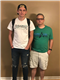# Additional Y Axis plotting incorrectly

Chart (HTML5)Top achievementsRank 2
Brian asked on 29 Nov 2014, 09:48 AM
I'm trying to plot a 2nd Y axis with the associated scale on the right of the graph.  When I plot the 2nd value though, it seems to be using the scale on the left.  What am I doing wrong here?  You can see from the attached graph snapshot that the plotting isn't what I'd expect.

`Dim` `lineMonthlyProfits ``As` `New` `LineSeries`
`Dim` `lineTotalProfits ``As` `New` `LineSeries`
`Dim` `yAxisTotalProfits ``As` `New` `AxisY`

`Do` `While` `ChartingMonth < Now()`
`    ``GrossProfit = 0 : Fees = 0`
`   ``'Code here that pulls values from db`
`    ``TotalProfit += GrossProfit - Fees`
`    ``lineMonthlyProfits.SeriesItems.Add(``CInt``(GrossProfit - Fees))`
`    ``lineTotalProfits.SeriesItems.Add(Int(TotalProfit))`
`    ``chartMonthlyProfits.PlotArea.XAxis.Items.Add(``CStr``(Month(ChartingMonth)) & ``"/"` `& Right(``CStr``(Year(ChartingMonth)), 2))`
`    ``ChartingMonth = DateAdd(DateInterval.Month, 1, ChartingMonth)`
`Loop`
`lineMonthlyProfits.Name = ``"Monthly Profit"`
`lineMonthlyProfits.LabelsAppearance.DataFormatString = ``"{0:c0}"`
`lineTotalProfits.LabelsAppearance.DataFormatString = ``"{0:c0}"`
`chartMonthlyProfits.PlotArea.Series.Add(lineMonthlyProfits)`
`chartMonthlyProfits.PlotArea.YAxis.MaxValue = 20000 ``'Not needed but in place for testing purposes`
`yAxisTotalProfits.Name = ``"Total Profits"`
`chartMonthlyProfits.PlotArea.AdditionalYAxes.Add(yAxisTotalProfits)`
`chartMonthlyProfits.PlotArea.XAxis.AxisCrossingPoints.Add(0)`
`chartMonthlyProfits.PlotArea.XAxis.AxisCrossingPoints.Add(lineTotalProfits.SeriesItems.Count)`
`lineTotalProfits.Name = yAxisTotalProfits.Name`
`chartMonthlyProfits.PlotArea.Series.Add(lineTotalProfits)`
`chartMonthlyProfits.PlotArea.YAxis.LabelsAppearance.DataFormatString = ``"{0:c0}"`
`chartMonthlyProfits.PlotArea.YAxis.MinorGridLines.Visible = ``False`
`chartMonthlyProfits.PlotArea.XAxis.MinorGridLines.Visible = ``False`
`chartMonthlyProfits.PlotArea.XAxis.MajorGridLines.Visible = ``False`
`chartMonthlyProfits.Legend.Appearance.Visible = ``False`

## 1 Answer, 1 is accepted

0Brian
Top achievementsRank 2
answered on 29 Nov 2014, 12:39 PM
I figured it out.  lineTotalProfits shoud use AxisName, not Name.
Tags
Chart (HTML5)Rank 2Rank 2